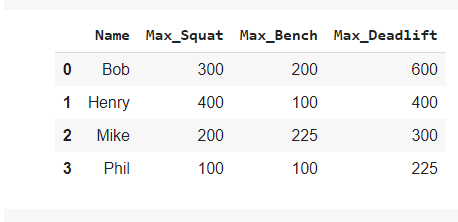# Need help passing in 2D Matrix to Conv1D layer and outputting a softmax probability

Hi how’s it going? I’m trying to build a model that takes in a 2D Matrix as a single sample and outputs the row index that’s the best action by using softmax.

This is what I have so far:

``````
names = ['Bob','Henry','Mike','Phil']

max_squat = [300,400,200,100]

max_bench = [200,100,225,100]

````````````
class Policy(nn.Module):

def __init__(self):
super(Policy, self).__init__()
self.layer1 = torch.nn.Conv1d(in_channels=4, out_channels=4, kernel_size=3, stride=1)

def forward(self, x):

x = self.layer1(x)
x = F.softmax(x,dim=1)

return x

def act(self, state):

state = state.float()
value = self.forward(state)

return value

policy = Policy()
result = policy.act(input_torch)

``````

The result shape is torch.Size([1,4,1]). How do I get this to output a column vector instead?

Also, is Conv1D with kernel size equal to number of features per row the most logical approach to this input state representation?